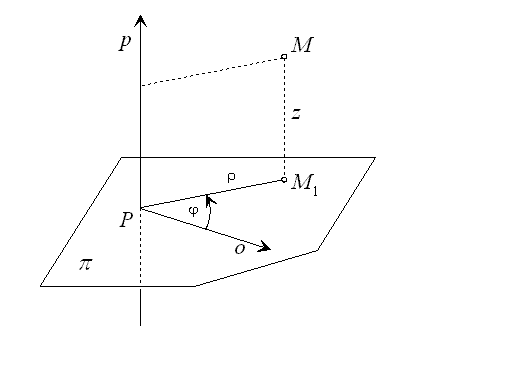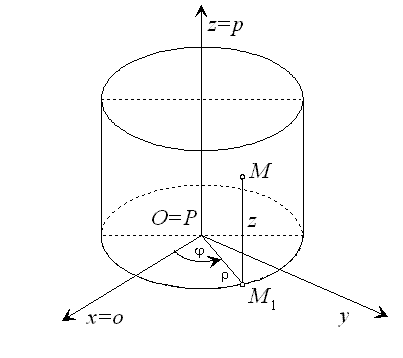# Cylindrical coordinates

Let the plane $\pi$ and perpendicular line p be given, while the polar coordinate system $\left(P,\phantom{\rule{2mm}{2mm}}\stackrel{\to }{o},\phantom{\rule{2mm}{2mm}}\varphi \right)$ be defined in plane $\pi$ such that the pole P be in the pierce point of plane $\pi$ and line p. Plane $\pi$ with the polar coordinate system and the coordinate axis p with the origin P form the cylindrical coordinate system $\left(P,\phantom{\rule{2mm}{2mm}}\stackrel{\to }{o},\phantom{\rule{2mm}{2mm}}\varphi ,\phantom{\rule{2mm}{2mm}}p\right)$ of the space.

Any point M in the space can be attached an ordered triple of real numbers, its cylindrical coordinates

 $M=\left(\rho ,\varphi ,z\right)$

such, that

1. $\rho ,\phantom{\rule{2mm}{2mm}}\varphi$ are polar coordinates of the orthographic view ${M}_{1}$ of the point M in the plane $\pi$ represented in the polar coordinate system $\left(P,\phantom{\rule{2mm}{2mm}}\stackrel{\to }{o},\phantom{\rule{2mm}{2mm}}\varphi \right)$,
2. z is the distance of point M from the plane $\pi \phantom{\rule{2mm}{2mm}}$and $\rho \in \left(0,\infty \right),\phantom{\rule{2mm}{2mm}}\varphi \in \left[0,2\pi \right)$, or $\varphi \in \left(-\pi ,\pi \right],\phantom{\rule{2mm}{2mm}}z\in \left(-\infty ,\infty \right)$.Figure 1: Cylindrical coordinate system

Pole P is the orthographic view in the plane $\pi$ of any point M located on the axis z, therefore cylindrical coordinates of all points on axis z are represented as triple $\left(0,\varphi ,z\right)$, where $\varphi$ is an arbitrary number.

Let the Cartesian coordinate system $\left(0,\phantom{\rule{2mm}{2mm}}x,\phantom{\rule{2mm}{2mm}}y,\phantom{\rule{2mm}{2mm}}z\right)$ and cylindrical coordinate system $\left(P,\phantom{\rule{2mm}{2mm}}\stackrel{\to }{o},\phantom{\rule{2mm}{2mm}}\varphi ,\phantom{\rule{2mm}{2mm}}z\right)$ be given. These two coordinate systems are called related (conjugate), iff

1. plane $\pi$ determining the cylindrical coordinate system $\left(P,\phantom{\rule{2mm}{2mm}}\stackrel{\to }{o},\phantom{\rule{2mm}{2mm}}\varphi ,\phantom{\rule{2mm}{2mm}}z\right)$ coincides with the plane xy of the Cartesian orthogonal coordinate system $\left(O,\phantom{\rule{2mm}{2mm}}x,\phantom{\rule{2mm}{2mm}}y,\phantom{\rule{2mm}{2mm}}z\right)\phantom{\rule{2mm}{2mm}}$
2. polar coordinate system $\left(P,\phantom{\rule{2mm}{2mm}}\stackrel{\to }{o},\phantom{\rule{2mm}{2mm}}\varphi \right)$ and the Cartesian orthogonal coordinate system $\left(O,\phantom{\rule{2mm}{2mm}}x,\phantom{\rule{2mm}{2mm}}y\right)$ in the plane $\pi$ are related (conjugate) systems
3. axis p in the cylindrical coordinate system $\left(P,\phantom{\rule{2mm}{2mm}}\stackrel{\to }{o},\phantom{\rule{2mm}{2mm}}\varphi ,\phantom{\rule{2mm}{2mm}}p\right)$ coincides with the coordinate axis z in the Cartesian orthogonal coordinate system $\left(O,\phantom{\rule{2mm}{2mm}}x,\phantom{\rule{2mm}{2mm}}y,\phantom{\rule{2mm}{2mm}}z\right)$.Figure 2: Relation between Cartesian and cylindrical coordinates

If $\left(x,y,z\right)$ are Cartesian coordinates and $\left(\rho ,\varphi ,z\right)$ are cylindrical coordinates of the point M not located on the axis z, their relation can be expressed by the following equations

 $x=\rho \mathrm{cos}\phantom{\rule{mediummathspace}{0.2em}}\varphi$
 $y=\rho \mathrm{sin}\phantom{\rule{mediummathspace}{0.2em}}\varphi$
 $z=z$

and since ${x}^{2}+{y}^{2}\ne 0$ and $\rho =\sqrt{{x}^{2}+{y}^{2}}$ also by equations

 $\varphi =\mathrm{arccos}\phantom{\rule{mediummathspace}{0.2em}}\frac{x}{\sqrt{{x}^{2}+{y}^{2}}},\phantom{\rule{2mm}{2mm}}y\ge 0$
 $\varphi =2\pi -\mathrm{arccos}\phantom{\rule{mediummathspace}{0.2em}}\frac{x}{\sqrt{{x}^{2}+{y}^{2}}},\phantom{\rule{2mm}{2mm}}y<0$

All points in the space with the constant first cylindrical coordinate, $\rho =a,\phantom{\rule{2mm}{2mm}}a>0$ are located on the circular cylindrical surface with the basic circle in the plane $\pi$. Centre of the circle is the pole P and radius equals a. Surface lines are parallel to the coordinate axis p, which is the axis of the cylindrical surface.